Impact Factor 3.560 | CiteScore 3.1
More on impact ›

# Frontiers in Physics## ORIGINAL RESEARCH article

Front. Phys., 07 May 2019 | https://doi.org/10.3389/fphy.2019.00065

# Rheology of High-Capillary Number Two-Phase Flow in Porous Media

• 1Beijing Computational Science Research Center, Beijing, China
• 2PoreLab, Department of Physics, Norwegian University of Science and Technology (NTNU), Trondheim, Norway
• 3PoreLab, Department of Physics, University of Oslo, Oslo, Norway

Flow of immiscible fluids in porous media at high capillary numbers may be characterized by an effective viscosity. We demonstrate that the effective viscosity is well-described by the Lichtenecker-Rother equation. Depending on the pore geometry, wettability, and viscosity of the fluids, the exponent α in this equation can have different values. We find α = 1 when fluids are well-mixed with small bubbles, α = 0.6 in two- and 0.5 in three-dimensional systems when there is less mixing with the appearance of big bubbles, and α = −0.5 when lubrication layers are formed along the pore walls. Our arguments are based on analytical and numerical methods.

## 1. Introduction

The hydrodynamics of real systems very often happens at small scale, such as in a porous medium . This is the case in a wide variety of biological, geological, and technological systems where there are often several immiscible fluids present. The challenge of describing such systems in a unified way, however, is largely unsolved. An important reason for this is the lack of a length scale above which the system may be averaged. Such a length scale gives rise to the representative elementary volume (REV) which is the conceptual basis for conventional theories that seek to up-scale the description of flow in porous media. However, since the fluid structures in question are often fractal, the REV average of intensive quantities, such as saturations, will depend on the size of the REV.

An important and rather general exception where this is not a problem, is the case of steady state flow [2, 3]. Steady state flow is characterized by potentially strong fluctuations at the pore scale, but with steady averages at the REV scale. Steady state configurations have much in common with ensembles in equilibrium statistical mechanics. Steady state flow implicitly assumed in conventional descriptions of porous media flows that take the existence of a REV for granted.

When the flow in question contains immiscible phases that are strongly forced in the sense that viscous forces dominate capillary forces, the description of the steady state simplifies to the description of a single fluid. This is the subject of the present work, and we show how the emergent description is manifestly incompatible with the conventional theories that have been in use for more than 80 years, most notably perhaps by the petroleum industry.

## 2. Theory

The first and still leading theory describing immiscible two-phase flow in porous media is that of Wyckoff and Botset . They based their theory of relative permeability on the idea that when the porous medium is seen from the viewpoint of one of the fluids, the pore volume accessible to this fluid would be the pore volume of the porous medium minus the pore volume occupied by the other fluid. This reduces the effective permeability seen by either fluid and the relative reduction factor is the relative permeability. In order to account for the surface tension between the immiscible fluids in the pores, the concept of capillary pressure was introduced . The central equations in relative permeability theory are

where the subscript j either refers to the wetting fluid (j = w) or the non-wetting fluid (j = n). ${\stackrel{\to }{v}}_{w}$ and ${\stackrel{\to }{v}}_{n}$ are superficial velocities of the two fluids, defined as the volumetric flow rates of each fluid entering a REV divided by the area of entry. K is the permeability of the porous medium, μw and μn are the wetting and non-wetting viscosities. kr,w(Sw) and kr,n(Sw) are the relative permeabilities and they are both functions of the wetting saturation Sw only. The corresponding non-wetting saturation is Sn = 1 − Sw. The wetting and non-wetting pressure fields Pw and Pn are related through the capillary pressure function Pc(Sw) = PnPw. We define a total superficial velocity $\stackrel{\to }{v}$ given by,

$\stackrel{\to }{v}$ is defined as the volumetric flow rate of all fluids entering the REV divided by the area of entry.

Let us now consider the case when the flow rates are so large that the capillary pressure may be ignored. Hence, we have Pn = Pw = P and we may combine the relative permeability Equation (1) with Equation (2) to find

where we have defined an effective viscosity μeff as

There have been many suggestions as to what functional form the relative permeabilities kr,w(Sw) and kr,n(Sw) take. The most common choice is to use those of Brooks and Corey assuming ${k}_{r,w}\left({S}_{w}\right)={k}_{r,w}^{0}{S}_{w}^{{n}_{w}}$ and ${k}_{r,n}\left({S}_{w}\right)={S}_{n}^{{n}_{n}}$ where $0\le {k}_{r,w}^{0}\le 1$ and the Corey exponents nw and nn being typically in the range 2–6 [6, 7].

Equation (4) is problematic. When μw = μn, a dependency of μeff on the saturation is predicted when nw and/or nn are larger than 1 when using the Brook–Corey relative permeabilities. Other functional forms for the relative permeabilities give similar dependencies. Clearly, such behavior is not physical.

McAdams et al. proposed an effective viscosity for two-phase flow by assuming a saturation-weighted harmonic average

Cicchitti et al. proposed an effective viscosity based on the saturation-weighted arithmetic average

Both of these expressions become saturation-independent when μw = μn as they should. There are several other proposals for the functional form of the effective viscosity μeff in the literature .

A one-dimensional porous medium, e.g., a capillary tube where the two fluids move as bubbles in series  constitutes a series coupling and the arithmetic average (6) is appropriate. If the capillary tubes forms a parallel bundle, each filled with either only the wetting or the non-wetting fluid, we have a parallel coupled system and Equation (5) is appropriate. We now consider a capillary bundle, where each capillary i in the bundle is filled with a bubble train with a corresponding wetting saturation Sw,i. The probability distribution for finding a capillary having this saturation, Sw,i, is p(Sw,i) so that

The capillary bundle is essentially a parallel combination of tubes, each filled with a series of bubbles. The effective viscosity for the capillary bundle is therefore given by,

As a model for the distribution p(Sw,i), we may take a Gaussian with a narrow width σ centered around Sw: $p\left({S}_{w,i}\right)=exp\left[-{\left({S}_{w,i}-{S}_{w}\right)}^{2}/2{\sigma }^{2}\right]/\sqrt{2\pi {\sigma }^{2}}$. Using this distribution for saturation we can integrate Equation (8) using a saddle point approximation and we find to order σ2 that,

We now consider a wide distribution of saturations in the capillaries. Considering a uniform distribution for p(Sw,i) in Equation (8) rather than a Gaussian, we find for an average wetting saturation Sw = 1/2,

The functional form of the latter equation is very different from the one for the Gaussian distribution, Equation (9).

For the extreme case when the capillaries are filled completely by either the wetting or the non-wetting fluids given by p(Sw,i) = Swδ(Sw,i − 1) + Snδ(Sw,i), we find the effective viscosity according to Equation (5), as already pointed out. We may study this either-or situation in a more complex network, namely a square lattice. We assume that the wetting saturation is set to Sw = 1/2, which defines the bond percolation threshold and that the links are randomly filled with either fluid. We may then use Straley's exact result  leading to an effective viscosity

We may calculate the effective viscosity of a regular lattice by using Kirkpatrick's mean field theory . The mobility between nodes i and j is Kijij where Kij is the permeability and μij is the effective viscosity of the link given by μij = μwSw,ijnSn,ij. Here Sw,ij and Sn,ij are the local wetting and non-wetting saturations in the links between the nodes. This form of μij is due to the fluids being connected in series in one link. Kirkpatrick's theory is based on the idea that the network with link mobilities Kijij may be replaced by a network with a uniform mobility Keff such that the total network mobility remains the same. In that case, the value of Keff is given by 

where z is the coordination number of the lattice. Considering the wetting saturation distribution p(Sw,ij) fulfilling Equation (7), the ensemble average is given by, $〈\dots 〉={\int }_{0}^{\infty }d{K}_{ij}P\left({K}_{ij}\right){\int }_{0}^{1}d{S}_{w,ij}p\left({S}_{w,ij}\right)\dots$, where P(Kij) is the permeability distribution. We assume a square lattice so that z = 4. By assuming that the saturation distribution is a narrowly peaked Gaussian with width σ, we may again use the saddle point approximation to get,

This is similar to that found for the parallel capillary bundle, Equation (9).

From the systems giving rise to Equations (9), (10), (11), and (13), the form of μeff is not clear. Does it depend on the details of the porous medium or is there a general form? We may generalize Equations (5) and (6) by writing them in the form

where α = −1 for parallel coupling and α = +1 for series coupling. Equation (14) has been used for estimating the effective electrical permittivity of heterogeneous conductors and in connection with permeability homogenization in porous media and is known as the Lichtenecker–Rother equation . The effective viscosity in (11) corresponds to α → 0, whereas Equations (9) and (13) suggest α = 1. Only Equation (10) does not fit this form.

In order to test Equation (14) in case of a porous medium, we now present two numerical approaches in the following: dynamic pore-network modeling and lattice Boltzmann simulations.

## 3. Pore-Network Modeling

The dynamic pore-network model used here has successfully explained several experimental and theoretical results for both the transient and steady-state two-phase flow in porous media over decades . During the transients, the model shows the different regimes of two-phase flow, namely the capillary fingering, viscous fingering, and the stable displacement pattern while changing the capillary number and viscosity ratio . In the steady state, the crossover from linear Darcy regime to a quadratic regime that was observed experimentally have also been studied with this pore-network model [19, 22]. The model have also shown the experimental observation of history independence in the steady-state two-phase flow at higher capillary numbers . Recently, relations between steady-state seepage velocities in porous media was obtained analytically by introducing of a new velocity function, the co-moving velocity. These relations were also established numerically with this model .

In the model, the porous medium is represented by a network of links, connected at nodes. In the links, two immiscible fluids, separated by interfaces, are transported. We consider both two-dimensional (2D) and three-dimensional (3D) networks for our simulations. For 2D, regular square and honeycomb networks with disordered link radii are used, whereas for 3D, reconstructed pore networks extracted from real samples are used . The flow rate inside a link between two neighboring nodes i and j with respective pressures pi and pj obeys

where lij is the link length and gij is the link mobility which is inversely proportional to the link viscosity given by μij = μwSw,ijnSn,ij [24, 25]. There is no contribution to the pressure from interfaces as the surface tension (γ) is zero. This sets the capillary number, defined as the ratio of viscous to capillary forces given by Ca = r/γ, to infinity. Here u is the Darcy velocity and μr is the viscosity of the more viscous fluid. Simulations are performed with a constant global pressure drop ΔP across the network and the local pressures (pi) are determined by solving the Kirchhoff equations. Flow rates qij through each link are then calculated using Equation (15) and the interfaces are moved with small time steps.

A crucial point here is how to distribute the two fluids after they mix at the nodes. Whether the system will allow high or low fragmentation of the fluids will depend on the geometry and nature of the pore space [26, 27]. This will have impact on the size of the bubbles and the number of interfaces inside a link. As small bubbles of either fluid may not necessarily imply a large number of interfaces or vice versa, we implemented two different algorithms for the interface dynamics. In the bubble-controlled algorithm, we decide the minimum size of a bubble before entering a link and in the interface-controlled algorithm we decide the maximum number of interfaces that can exist in a link. We considered two different possibilities for each algorithm: for the bubble-controlled case, (A) small bubbles are allowed, with minimum sizes bmin = 0.02rij, (B) bubbles with sizes at least equal to the respective pore radii (bmin = rij) are allowed. For the interface-controlled algorithm, we study two cases, (C) one with maximum four and (D) another with maximum two interfaces per link. Our model does not include lubrication layers, and the simulations therefore cannot capture the wetting film effects at the pore walls. More details of the interface algorithm is provided in the Supplementary Material.

## 4. Lattice Boltzmann Simulations

We then turn to lattice Boltzmann simulations which have no explicit parameters for the bubble size or for the number of interfaces and permits arbitrary shapes of the fluid domains within the link. The lattice Boltzmann model applied here is based on the original triangular lattice and the interaction rules first introduced by Gunstensen et al. . It models the Navier–Stokes equation for two immiscible fluids within a 2D pore geometry of rectangular pipes of equal width, and in the pores the fluids organize only according to the flow and geometry of the system. The two fluids are represented by different colors, here red (more viscous) and blue (less viscous), and their respective densities ρr and ρb define a local color gradient. The surface tension is introduced by the application of two steps, first a perturbation of the mass distribution that is proportional to the magnitude of the color gradient, thus increasing the mass in the directions transverse to a fluid-fluid interface, and second, a re-coloring step that sends red toward red and blue toward blue. Both steps conserve the local momentum, the first step creates the change in the stress tensor which is responsible for the surface tension, and the last step causes an anti-diffusive flux of both phases. The solid obstacles are represented by the bounce-back rule, which ensures the hydrodynamic no-slip condition and the wetting property is controlled by coloring the solid obstacles with the same saturations as in the bulk fluid. The aim is to simulate flows that are not governed by surface tension effects and this wetting rule creates a relatively neutral wetting property that does not affect the flow as much as full wetting of one phase. The model also allows for tuning of the surface tension γ, so that the capillary number given by $\mathrm{\text{Ca}}=\frac{u{\mu }_{r}}{\gamma }$, is set to high values. Here, u is the overall Darcy velocity and μr is the viscosity of the red fluid with higher viscosity. In all the simulations Ca>9. For the more viscous wetting fluid, the wetting saturation Sw = ρr/(ρrb) controls the viscosity according to the local rule

where M = μbr here and the pressure gradient is implemented as a constant body force in the diagonal direction point to upper right corner of the simulation domain. The body force is introduced as a constant momentum input at every time step and at every lattice site.

Initially, the flow velocity is zero everywhere and ρr and ρb initialized according to the specified value of Sw but with a small random component added. This randomness then triggers an initial phase separation which is responsible for the subsequent distribution of bubbles. Unlike the network modeling, the wetting effects of the pore walls are included here . For the neutral wetting condition and for more viscous wetting fluid, we choose a rectangular pore network to emulate the network model. For the case of complete wetting with less viscous wetting fluid, the wetting layers are important and we therefore avoid the singular sharp corners. The model is implemented on a 128 × 128 biperiodic lattice with the pressure gradient implemented as a constant body force in the diagonal direction pointing to the upper right corner.

## 5. Results and Discussion

We perform simulations under constant external pressure drop ΔP and the systems are evolved to the steady state. The results here are in the high capillary number regime and therefore do not depend on the history or the initial preparation of the system . In the steady state, we compare the results with ${\left({\mu }_{\text{eff}}/{\mu }_{w}\right)}^{\alpha }={S}_{w}+{M}^{\alpha }{S}_{n}$ (Equation 14), where M = μnw. In the network model, we measure the total flow rate Q as a function of the saturations Sw. As $Q=-\frac{KA}{L{\mu }_{\text{eff}}}\Delta P$, we measure μeffw by calculating Qw/Q where Qw is the total flow rate at Sw = 1. In the lattice Boltzmann simulations, the μeff is calculated by measuring the effective permeability, obtained by measuring the total flux Q through the system and dividing by the forcing or average pressure gradient. We chose M = 2, 5, and 10 here. Higher values of M increase the computational cost and do not change the conclusions of this study for the network model with γ = 0. Simulations with M and 1/M produce the same results due to symmetric bubble rules and the absence of film flow in the network model. Depending on the pore geometry, wettability and viscosities of the fluids, we find three flow regimes. All can be characterized by Equation (14) with three different values of α. When smaller bubbles (model A) or more interfaces (model C) are allowed in the network model, we find α = 1 for both 2D and 3D systems as shown in Figures 1, 2, respectively were the fluids are well mixed. This regime is also observed in the lattice Boltzmann simulations for neutral wetting properties, or when the wetting fluid is more viscous. This is shown in Figure 3, where the straight lines confirm α = 1 in Equation (14). Here the continuous merging and break-up of droplets give rise to a flow where each pore channel contains a sequence of individual drops. The fluids effectively behave as if they are arranged in series, and on the average the life-time of the droplets does not have any impact on the up-scaled behavior.

FIGURE 1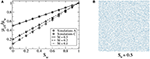Figure 1. (A) Plot of ${\left({\mu }_{\mathrm{\text{eff}}}/{\mu }_{\mathrm{\text{w}}}\right)}^{\alpha }$ obtained from 2D network simulations (symbols) with small bubbles (A) or with many interfaces (C). Results are consistent with Equation (14) (straight lines) with α = 1. A steady-state snapshot for model C is shown in (B), where gray and blue are the wetting and non-wetting fluids respectively. Here the wetting fluid is more viscous and the results for less viscous wetting fluid are the same for the network model due to the symmetry in the interface rules and the lack of film flow mechanism in the model.

FIGURE 2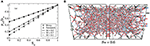Figure 2. (A) Plot of ${\left({\mu }_{\mathrm{\text{eff}}}/{\mu }_{\mathrm{\text{w}}}\right)}^{\alpha }$ for 3D networks reconstructed from Berea sandstone and sandpack samples for the simulations with four interfaces (C). Results are consistent with Equation (14) (straight lines) with α = 1, similar to the 2D networks. A snapshot of fluids in Berea sandstone in the steady state for model C is shown in (B), where blue and red are the wetting and non-wetting fluids respectively.

FIGURE 3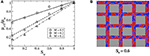Figure 3. (A) Plot of ${\left({\mu }_{\mathrm{\text{eff}}}/{\mu }_{\mathrm{\text{w}}}\right)}^{\alpha }$ obtained from lattice Boltzmann simulations with more viscous wetting fluid which shows α = 1 when compared with Equation (14). (B) Typical steady-state distribution of the fluids, where the blue and red are the more viscous (wetting) and less-viscous (non-wetting) fluids, respectively.

When we allow only larger bubbles with the size of the order of the pore size (model B) or few interfaces (model D) in the network model, we find α = 0.6 for 2D and α = 0.5 for 3D that are consistent with Equation (14). Results are plotted in Figures 4, 5, respectively. Here the steady-state fluid distribution shows less mixing and larger clusters compared to Figure 1. This also affects the fractional flow, making the less viscous fluid to flow with higher velocity (Supplementary Material). So far, we could not find a set of suitable parameters or pore geometry for the lattice Boltzmann simulations that can reproduce this regime of flow.

FIGURE 4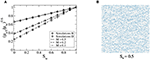Figure 4. (A) Plot of ${\left({\mu }_{\mathrm{\text{eff}}}/{\mu }_{\mathrm{\text{w}}}\right)}^{\alpha }$ obtained from network simulations with larger bubbles (B) or few interfaces (D) which shows α = 0.6 for 2D. (B) Typical steady-state snapshot for model D, showing less mixing of fluids and larger clusters compared to Figure 1B.

FIGURE 5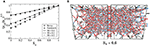Figure 5. (A) Plot of ${\left({\mu }_{\mathrm{\text{eff}}}/{\mu }_{\mathrm{\text{w}}}\right)}^{\alpha }$ for 3D networks reconstructed from Berea sandstone and sandpack samples for the simulations with two interfaces (D). Results are consistent with Equation (14) (straight lines) with α = 0.5. A steady-state snapshot of Berea sandstone for model D is shown in (B), where blue and red are the wetting and non-wetting fluids respectively.

When the wetting fluid is made less viscous in the lattice Boltzmann simulations, it produces lubrication layers of the wetting fluid along the pore walls. This introduces a third regime with a negative value of α. The results are shown in Figure 6 which indicate a robust α = −0.5 behavior over a range of M values. This means that, due to the lubrication layers flow comes close to the parallel-coupling scenario, which is described by α = −1, but there is still a significant difference. The flow paths that appear in parallel are not stationary as they would be in a parallel coupled system, they break up and merge continuously. We could not study this regime with our network model as the model does not contain film flow. It will be interesting to study this in the future with a network model that includes the film flow .

FIGURE 6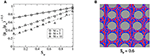Figure 6. (A) Plot of ${\left({\mu }_{\mathrm{\text{eff}}}/{\mu }_{\mathrm{\text{w}}}\right)}^{\alpha }$ from lattice Boltzmann simulations with less viscous wetting fluid where we find α = −0.5. The steady state is dominated by lubrication layers of less viscous blue fluid as seen in (B). The end points close to Sw = 1 fall a little below 1, which could have several explanations, one being finite Reynolds numbers, an effect that is likely to increase with increasing M the way the simulations are done.

## 6. Conclusion

In summary, we show that immiscible two-phase flow in porous media at high capillary number limit can be characterized by measuring the effective viscosity in the steady state. We find that the Lichtenecker–Rother Equation (14) describes the effective viscosity well for different flow configurations. We identified three flow regimes characterized by the exponent α, which depend on the organization of the two fluids in the pores. When the fluids are well mixed, we find a result which is consistent with the Kirkpatrick's mean field theory  with α = 1. This is observed in both the network model and lattice Boltzmann simulations, by allowing small bubbles or more interfaces in the network model, and with the neutral wetting condition or more viscous wetting fluid in the lattice Boltzmann simulations. When only larger bubbles or fewer interfaces are allowed, we find the second regime with α = 0.6 in 2D and α = 0.5 in 3D with the network model. Third, when the wetting fluid is less viscous, lubrication layers are formed at the pore walls, and we find α = −0.5 from the lattice Boltzmann simulations.

Finally, we like to point out that in the network model, we have varied the minimum bubble size over the range 0.02rij to 0.5rij finding α decreasing gradually from 1 to 0.6. Taking into account that rij ≤ 0.4l, where l is the link length, this shift of α from 1 to 0.6 occurs over the narrow range from 0.008l to 0.2l, indicating that we see a crossover. In case of the lattice Boltzmann simulation there is no gradual transition with different wetting properties from α = 1 to α = −0.5. The former is observed in the neutrally wetting case or in the case when the viscous fluid is the completely wetting. The latter is observed in the case of complete wetting of the less viscous fluid.

## Author Contributions

MG and SS did the network model computations. EF did the Lattice Boltzmann computations. AH wrote the first draft of the manuscript. All the authors contributed in developing the theory and developing the manuscript to its final form.

## Conflict of Interest Statement

The authors declare that the research was conducted in the absence of any commercial or financial relationships that could be construed as a potential conflict of interest.

## Acknowledgments

The authors thank Dick Bedeaux, Carl Fredrik Berg, Signe Kjelstrup, Knut Jørgen Måløy, Per Arne Slotte, and Ole Torsæter for interesting discussions. EF and AH thank the Beijing Computational Science Research Center (CSRC) and Hai-Qing Lin for hospitality. SS was supported by the National Natural Science Foundation of China under grant number 11750110430. This work was partly supported by the Research Council of Norway through its Centers of Excellence funding scheme, project number 262644.

## Supplementary Material

The Supplementary Material for this article can be found online at: https://www.frontiersin.org/articles/10.3389/fphy.2019.00065/full#supplementary-material

## References

1. Bear J. Dynamics of Fluids in Porous Media. Mineola, NY: Dover (1988).

2. Tallakstad KT, Knudsen HA, Ramstad T, Løvoll G, Måløy KJ, Toussaint R, et al. Steady-state two-phase flow in porous media: statistics and transport properties. Phys Rev Lett. (2009) 102:074502. doi: 10.1103/PhysRevLett.102.074502

3. Aursjø O, Erpelding M, Tallakstad KT, Flekkøy EG, Hansen A, Måløy KJ. Film flow dominated simultaneous flow of two viscous incompressible fluids through a porous medium. Front Phys. (2014) 2:63. doi: 10.3389/fphy.2014.00063

4. Wyckoff RD, Botset HG. The flow of gasliquid mixtures through unconsolidated sands. J Appl Phys. (1936) 7:325. doi: 10.1063/1.1745402

5. Leverett MC. Capillary behavior in porous solids. Trans AIME. (1941) 142:152. doi: 10.2118/941152-G

6. Brooks RH, Corey AT. Hydraulic properties of porous media. In: Corey AT, Dils RE and Yevdjevich VM, editors. Hydrology Papers 3. Fort Collins, CO: Colorado State University (1964). p. 27.

7. Lake LW. Enhanced Oil Recovery. Englewood Cliffs, NJ: Prentice Hall (1989).

8. McAdams WH, Woods WK, Heroman LC Jr. Vaporization inside horizontal tubes-II: Benzene-oil mixtures. Trans ASME. (1942) 64:193.

9. Cicchitti A, Lombardi C, Silvestri M, Soldaini G, Zavattarelli R. Two-phase cooling experiments: Pressure drop, heat transfer and burnout measurements. Energia Nucl. (1960) 7:407.

10. Awad M, Muzychka YS. Effective property models for homogeneous two-phase flows. Exp Therm Fluid Sci. (2008) 33:106. doi: 10.1016/j.expthermflusci.2008.07.006

11. Sinha S, Hansen A, Bedeaux D, Kjelstrup S. Effective rheology of bubbles moving in a capillary tube. Phys Rev E. (2013) 87:025001. doi: 10.1103/PhysRevE.87.025001

12. Straley JP. Critical exponents for the conductivity of random resistor lattices. Phys Rev B. (1977) 15:5733. doi: 10.1103/PhysRevB.15.5733

13. Kirkpatrick S. Percolation and conduction. Rev Mod Phys. (1973) 45:574. doi: 10.1103/RevModPhys.45.574

14. Lichtenecker K, Rother K. Die Herleitung des logarithmischen Mischungsgesetzes aus allgemeinen Prinzipien der stationären Strömung. Phys Z. (1931) 32:255.

15. Guéguen Y, Palciauskas V. Introduction to the Physics of Rocks. Princeton, NJ: Princeton University Press (1994).

16. Todd MG, Shi FG. Complex permittivity of composite systems: a comprehensive interphase approach. IEEE Trans Dielectr Electr Insul. (2005) 12:601. doi: 10.1109/TDEI.2005.1453466

17. Brovelli A, Cassiani C. A combination of the Hashin-Shtrikman bounds aimed at modelling electrical conductivity and permittivity of variably saturated porous media. Geophys J Int. (2010) 180:225. doi: 10.1111/j.1365-246X.2009.04415.x

18. Aker E, Måløy KJ, Hansen A, Batrouni GG. A two-dimensional network simulator for two-phase flow in porous media. Transp Porous Media. (1998) 32:163. doi: 10.1023/A:1006510106194

19. Sinha S, Bender AT, Danczyk M, Keepseagle K, Prather CA, Bray JM, et al. Effective rheology of two-phase flow in three-dimensional porous media: Experiment and simulation. Transp Porous Media. (2017) 119:77. doi: 10.1007/s11242-017-0874-4

20. Erpelding M, Sinha S, Tallakstad KT, Hansen A, Flekkøy EG, Måløy KJ. History independence of steady state in simultaneous two-phase flow through two-dimensional porous media. Phys Rev E. (2013) 88:053004. doi: 10.1103/PhysRevE.88.053004

21. Gjennestad MA, Vassvik M, Kjelstrup S, Hansen A. Stable and efficient time integration of a dynamic pore network model for two-phase flow in porous media. Front Phys. (2018) 13:56. doi: 10.3389/fphy.2018.00056

22. Sinha S, Hansen A. Effective rheology of immiscible two-phase flow in porous media. Europhys Lett. (2012) 99:44004. doi: 10.1209/0295-5075/99/44004

23. Hansen A, Sinha S, Bedeaux D, Kjelstrup S, Gjennestad MA, Vassvik M. Relations between seepage velocities in immiscible, incompressible two-phase flow in porous media. Transp Porous Media. (2018) 125:565. doi: 10.1007/s11242-018-1139-6

24. Langglois WE. Slow Viscous Flow. New York, NY: The Macmillan Company (1964).

25. Jia P, Dong M, Dai L, Yao J. Slow viscous flow through arbitrary triangular tubes and its application in modelling porous media flows. Transp Porous Media. (2008) 74:153. doi: 10.1007/s11242-007-9187-3

26. Ody CP, Baroud CN, de Langre E. Transport of wetting liquid plugs in bifurcating microfluidic channels. J Colloid Interface Sci. (2007) 308:231. doi: 10.1016/j.jcis.2006.12.018

27. Liu X, Zhang C, Yu W, Deng Z, Chen Y. Bubble breakup in a microfluidic T-junction. Sci Bull. (2016) 61:811. doi: 10.1007/s11434-016-1067-1

28. Gunstensen AK, Rothman DH, Zaleski S, Zanetti G. Lattice Boltzmann model of immiscible fluids. Phys Rev A. (1991) 43:4320. doi: 10.1103/PhysRevA.43.4320

29. Rothman DH, Zaleski S. Lattice-Gas Cellular Automata: Simple Models of Complex Hydrodynamics. Cambridge: Cambridge University Press (2004).

30. Tørå G, Øren PE, Hansen A. A dynamic network model for two-phase flow in porous media. Transp Porous Media. (2012) 92:145. doi: 10.1007/s11242-011-9895-6

Keywords: porous media, two-phase flow, effective viscosity, pore-network modeling, lattice-boltzman method (LBM)

Citation: Sinha S, Gjennestad MA, Vassvik M, Winkler M, Hansen A and Flekkøy EG (2019) Rheology of High-Capillary Number Two-Phase Flow in Porous Media. Front. Phys. 7:65. doi: 10.3389/fphy.2019.00065

Received: 11 January 2019; Accepted: 15 April 2019;
Published: 07 May 2019.

Edited by: